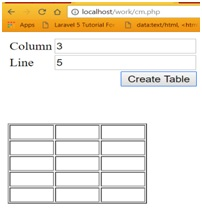# How to create tables dynamically from user input?

Here, we will learn how to generate a table dynamically using PHP code/script by passing number of rows and columns through form?
Submitted by IncludeHelp, on October 15, 2017 [Last updated : March 12, 2023]

## Creating tables dynamically in PHP

By executing this code, we need to enter number of rows (line) and number of columns through HTML form and then PHP script/code will generate the table dynamically according to the given input values.

## PHP code to create tables dynamically from user input

Here is the PHP Code/Script to generate table randomly,

```<?php
\$crtable = "";
if (\$_POST) {
\$crtable .= '<table border="1">';
for (\$i = 0; \$i < \$_POST["line"]; \$i++) {
\$crtable .= "<tr>";
for (\$j = 0; \$j < \$_POST["colunn"]; \$j++) {
\$crtable .= '<td width="50">&nbsp;</td>';
}
\$crtable .= "</tr>";
}
\$crtable .= "</table>";
}
?>
<form action="" method="post">
<table border="0" width="200">
<tr>
<td width="80"><label>Column:</label></td>
<td width="120"><input type="text" name="colunn"></td>
</tr>
<tr>
<td><label>Line:</label></td>
<td><input type="text" name="line"></td>
</tr>
<tr>
<td colspan="2" align="right">
<input type="submit" value="Create Table">
</td>
</tr>
</table>
</form>
<br/>
<br/>

<?php echo \$crtable;
?>
```

## Output